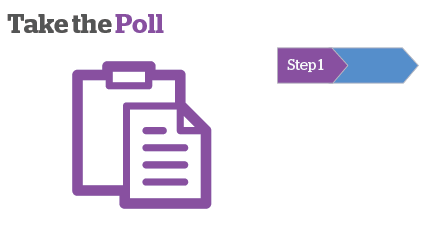Q. How should Yield be calculated? A. Annual Rental Income Divided by Purchase Price Multiplied by 100 B. Annual Rental Income Less expenses divided by Mortgage Value Multiplied by 100 C. Annual Rental Income Less expenses divided by Cost of deal[your personal capital] invested, Multiplied by 100 D. Annual Rental Income Less expenses[interest element only] divided by Capital invested Multiplied by 100 Q. 2012 are house prices likely to? A. Rise By More than 3% A. Decline By More than 3% C. Remain Static